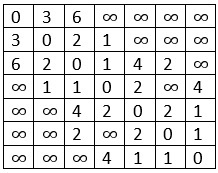# Dijkstra’s Shortest Path Algorithm

The main problem is the same as the previous one, from the starting node to any other node, find the smallest distances. In this problem, the main difference is that the graph is represented using the adjacency matrix. (Cost matrix and adjacency matrix is similar for this purpose).

For the adjacency list representation, the time complexity is O(V^2) where V is the number of nodes in the graph G(V, E)

## Input and Output

Input:Output:
0 to 1, Using: 0, Cost: 3
0 to 2, Using: 1, Cost: 5
0 to 3, Using: 1, Cost: 4
0 to 4, Using: 3, Cost: 6
0 to 5, Using: 2, Cost: 7
0 to 6, Using: 4, Cost: 7

## Algorithm

dijkstraShortestPath(n, dist, next, start)

Input − Total number of nodes n, distance list for each vertex, next list to store which node comes next, and the seed or start vertex.

Output − The shortest paths from start to all other vertices.

Begin
create a status list to hold the current status of the selected node
for all vertices u in V do
status[u] := unconsidered
dist[u] := distance from source using cost matrix
next[u] := start
done

status[start] := considered, dist[start] := 0 and next[start] := φ
while take unconsidered vertex u as distance is minimum do
status[u] := considered
for all vertex v in V do
if status[v] = unconsidered then
if dist[v] > dist[u] + cost[u,v] then
dist[v] := dist[u] + cost[u,v]
next[v] := u
done
done
End

## Example

#include<iostream>
#define V 7
#define INF 999
using namespace std;

// Cost matrix of the graph
int costMat[V][V] = {
{0, 3, 6, INF, INF, INF, INF},
{3, 0, 2, 1, INF, INF, INF},
{6, 2, 0, 1, 4, 2, INF},
{INF, 1, 1, 0, 2, INF, 4},
{INF, INF, 4, 2, 0, 2, 1},
{INF, INF, 2, INF, 2, 0, 1},
{INF, INF, INF, 4, 1, 1, 0}
};

int minimum(int *status, int *dis, int n) {
int i, min, index;
min = INF;

for(i = 0; i<n; i++)
if(dis[i] < min && status[i] == 1) {
min = dis[i];
index = i;
}

if(status[index] == 1)
return index; //minimum unconsidered vertex distance
else
return -1;    //when all vertices considered
}

void dijkstra(int n, int *dist,int *next, int s) {
int status[V];
int u, v;

//initialization
for(u = 0; u<n; u++) {
status[u] = 1;               //unconsidered vertex
dist[u] = costMat[u][s];     //distance from source
next[u] = s;
}

//for source vertex
status[s] = 2; dist[s] = 0; next[s] = -1; //-1 for starting vertex

while((u = minimum(status, dist, n)) > -1) {
status[u] = 2;//now considered
for(v = 0; v<n; v++)
if(status[v] == 1)
if(dist[v] > dist[u] + costMat[u][v]) {
dist[v] = dist[u] + costMat[u][v];   //update distance
next[v] = u;
}
}
}

main() {
int dis[V], next[V], i, start = 0;
dijkstra(V, dis, next, start);

for(i = 0; i<V; i++)
if(i != start)
cout << start << " to " << i <<", Using: " << next[i] << ",
Cost: " << dis[i] << endl;
}

## Output

0 to 1, Using: 0, Cost: 3
0 to 2, Using: 1, Cost: 5
0 to 3, Using: 1, Cost: 4
0 to 4, Using: 3, Cost: 6
0 to 5, Using: 2, Cost: 7
0 to 6, Using: 4, Cost: 7